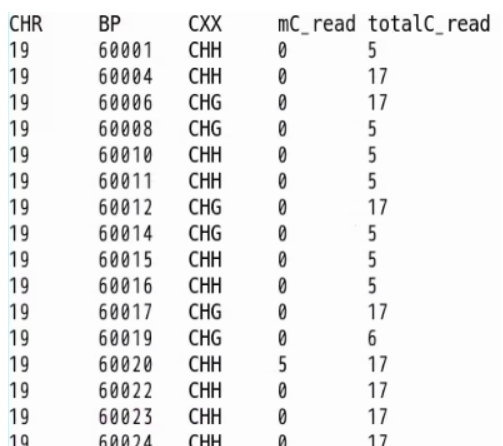## CpGCpHhMM

### hidden Markov model for detecting CpG-CpH differentially methylated regions

To run CpGCpHhMM,

[vlog]

### IntroductionMethylated non-CpGs (mCpHs) in mammalian cells yield weak enrichment signals and colocalize with methylated CpGs (mCpGs). The mCpHs are cell type-specific and associated with epigenetic regulation, although their dependency on mCpGs remains to be elucidated.We developed a hidden Markov model (HMM) to systematically detect genomic regions in which CpG and CpH are differentially methylated, providing an opportunity to infer the functional importance of non-CpG methylation.
An empirical HMM was designed to detect the differentially methylated regions (DMRs) of CpG and CpH (CpG-CpH DMRs) in each human sample. Specifically, the whole genome was segmented into 180 bp-long bins and the emission probability E for a state {P, N, or U) at each bin was calculated; P, positive correlation between CpH and mCpG methylation; N, negative correlation between CpH and CpG methylation; U, uncorrelated. The E for bins in which the number of reads aligned at CpGs and CpHs was >10 was calculated. In addition, to ensure the continuity of the Markov model, the genomic region was divided if the continuous undetected bins were longer than 100,000 bp, and the HMM was applied separately. The probability of state transition was estimated using an expectation-maximization (EM) algorithm that repeats the EM steps until the difference between the previous and current transition probabilities of all state transactions is <5e-4. Then, the Viterbi algorithm that finds an optimal path among the states was applied, and the bins were re-defined to P-, N-, or U-state. The consecutive bins were linked if they were in the same state and the distance between them was <3 bins (540 bp). Finally, the N-state regions were defined as CpG–CpH DMRs. More information is avaiable at Lee et al (2020).### Instruction

Input file
• GZIP file with tap-seperated 4 columns.
CHR: chromosome number (1..19,X,Y)
BP: position of the cytosine
CXX: cytosine context. CG, CHH, or CHG (both CHH and CHG are recognized as CH)
• Example:
19 60001 CHH 0 10
19 60006 CHG 0 17
19 60120 CG 2 2
...

• Output
• output_dir/gblock/
pos: starting position of 180bp bin
mCGn: sum of methylated read count aligned at CG in the bin
CGn: sum of total read count aligned at CG in the bin
mCHn: sum of methylated read count aligned at CH in the bin
CHn: sum of total read count aligned at CH in the bin
refCGn: number of CG in the bin in hg19
refCHn: number of CH in the bin in hg19

• output_dir/emit/
pos: starting position of 180bp bin
mCGlv: average methylation level at CG
refCGn: number of CG in the bin in hg19
mCHlv: average methylation level at CH
refCHn: number of CH in the bin in hg19
e_p: probability that the bin is belong to P-state
e_n: probability that the bin is belong to N-state
e_i: probability that the bin is belong to I-state
max_stat: state of top probability (0:P, 1:N, 2:I)

• output_dir/initProb/
randomly set initial probability of P-, N-, and I-state

• output_dir/TRN/
log-scaled transition rates between states. column order: P, N, I, row order: P, N, I

• output_dir/viterbi/
pos: starting position of 180bp bin
mCGlv: average methylation level at CG
refCGn: number of CG in the bin in hg19
mCHlv: average methylation level at CH
refCHn: number of CH in the bin in hg19
state: state designated by Viterbi decoding (0:P-, 1:N-, 2:I-state)

• output_dir/statistics/
Number of bins deteced as P, N, and I-state by top emission probability and Viterbi decoding

• NOTE:
• n/a

• Reference
1. Jong-Hun Lee, Yutaka Saito, Sung-Joon Park, Kenta Nakai, "Existence and possible roles of independent non-CpG methylation in the mammalian brain", DNA Research dsaa020 (2020)